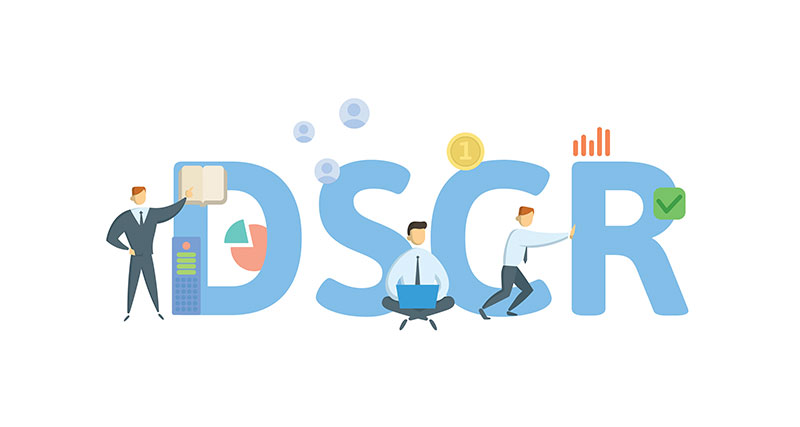## How Interest Rates Are Impacting the Debt Service Coverage RatioChris Peters
April 22, 2022
4
minThe recent increase in interest rates has given everyone heartburn, even the folks here at StackSource. While the pandemic brought about a once in a generation opportunity to lock in financing at historic lows, rates have increased back up to more normal levels, with the 10-year US Treasury breaching 2.75% as I write this blog post.

So, other than increasing the cost of debt financing, how do increased interest rates impact the overall financing package? One impact we are seeing is when lenders use DSCR (Debt Service Coverage Ratio) to size a loan.

Lenders use DSCR to ensure that the NOI (income after paying operating expenses) covers annual debt service (principal and interest) by some margin, typically 1.25x. That means that if there is \$100,000 of annual debt service, NOI needs to be \$125,000 (\$100,000 x 1.25 = \$125,000). The higher the NOI compared to the annual debt service, the greater the DSCR and the more likely a lender will fund that loan.

Now that rates are coming off historic lows, lenders are using higher interest rates when they calculate their annual debt service. Let’s see how these higher rates will increase debt service and ultimately impact DSCR.

Let’s assume a property has a \$10,000,000 purchase price and NOI of \$600,000. We can infer the cap rate is 6.00% (\$600,000 / \$10,000,000 = 6.00%). Assuming a lender has a minimum DSCR of 1.25x, the max annual debt service will be \$480,000 (\$600,000 / 1.25x = \$480,000). Lenders will then back into the max loan amount using their current interest rate and amortization. Now, let’s roll this back 6-12 months ago when interest rates were much lower and assume the rate is 3.50% and we have a 25 year amortization. With debt service of \$480,000, an 3.50% interest rate, and a 25-year amortization, the max loan amount would be \$7,990,036, which comes out to about 79.90% LTV based on the \$10,000,000 purchase price.

Below is an excel calculation in which we solved for the Max Loan Amount. I’ve included the formula below as well.

Max Loan Amount = 1-PV(rate/12,amortization*12,(NOI/12)/DSCR)

Now if we assume rates have increased to 4.75% in today’s rate environment and we keep the loan amount the same and all other factors the same, the annual debt service would be \$546,631 and would cause our DSCR to fall from 1.25x to 1.10x.

So, a 1.25% increase in interest rate caused our annual debt service to increase 66,631 which then caused our DSCR to drop to 1.10x, well below the 1.25x threshold for most lenders.

So, in order to meet that 1.25x DSCR at the new increased interest rate, lenders will decrease the loan amount so that the debt service also drops low enough that the DSCR increases to 1.25x.

If we use the 4.75% interest rate and keep all other factors the same but adjust the loan amount lower to decrease the annual debt service, the max loan amount will be \$7,016,100 in order to arrive at the \$480,000 annual debt service and meet our 1.25x DSCR. This new loan amount comes out to about 70.16% LTV.

By increasing the interest rate and keeping all other factors constant, the 1.25% increase in interest rate (4.75% vs. 3.50%) will need to be offset by a decrease in loan proceeds of \$973,936 (\$7,990,036 vs. \$7,016,100) or nearly 10% of the total purchase price.

When sizing a loan, lenders are having difficulties determining where rates will be in 45-60 days when the deal will actually close so don’t be surprised if lenders err on the higher end of the rate range in anticipation of further increasing interest rates.Find the right commercial real estate financing with StackSource by getting instantly matched to the best debt and equity options for your project from our nationwide network of capital sources.